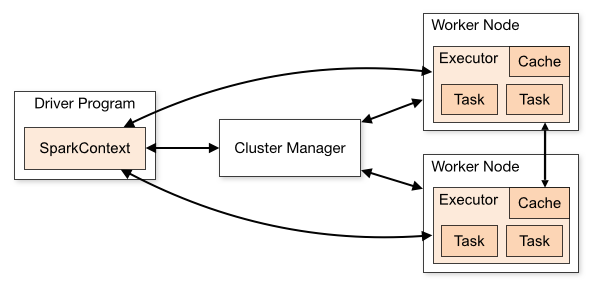In this post, I describe how to parallelize computations in Ruby with ruby-spark gem. This library uses a Apache Spark project to storing and distributing data collections across the cluster.

Requirments:

• Java 7+
• Ruby 2+
• wget or curl
• MRI or JRuby

Glossary:

• Context: entry point for using Spark functionality
• RDD: Resilient Distributed Dataset
• Driver: a driver Spark instance (exist only once)
• Executor: worker instance## Installation

``````# Install gem
gem install ruby-spark

# Build Spark and extensions (could take a while)
ruby-spark build

# Set JAVA_HOME (required for MRI)
export JAVA_HOME="..."``````

## Starting and configurations

For all setup options, please look on wiki. All necessary configuration are set by default but if you want change it you need set keys before creating context. After that is configuration read-only.

``````require 'ruby-spark'

# Configuration
Spark.config do
set_app_name 'My RubySpark'
set_master   'local[*]'
set 'spark.ruby.serializer',           'marshal'
set 'spark.ruby.serializer.batch_size', 2048
end

# Create a context
Spark.start

# Context reference
sc = Spark.sc``````

You can also start prepared console by `ruby-spark shell`. This command will load RubySpark and create Pry console.

## Usage

### Creating RDD

First, you need create a distributed data collection. This dataset will be splitted into computing process. All process have the same computing function and cannot comunicate with each other.

``````worker_nums = 2
rands = Array.new(1000){ rand(1..10) }

rdd_numbers = sc.parallelize(1..1000, worker_nums)
rdd_rands = sc.parallelize(rands, worker_nums)
text_file = sc.text_file('/etc/hosts', worker_nums)``````

### Custom serializer

RDD is using by default serializer defined from confing options (`spark.ruby-serializer*`). However if you want a different serializer just for one RDD you can do:

``````ser = Spark::Serializer.build { auto_batched(compressed(oj)) }
custom_rdd = sc.parallelize(1..1000, worker_nums, ser)``````

This can be useful for different data types. For example oj is really faster but serialized objects can be very large.

### Examples

Now you can define a computing function. All function can be found at Rubydoc or . Every new function is attached to the RDD and are executed at once by `.collect` (lazy definition).

Methods can be divided into:

• Transformations:
`.map, .flat_map, .map_partitions, .filter, .compact, .glom, .distinct, .shuffle, ...`
• Actions: (calculation is started immediately)
`.take, .first, .aggregate, .max, .min, .sum, ...`

#### Simple mapping

This function will be applied to every element in the collection.

``````rdd_x2 = rdd_numbers.map(lambda{|x| x*2})
rdd_x2.collect # => [2, 4, 6, 8, 10, 12, ...]``````

#### Pipelined functions

You can also add new function to old RDD.

``````filtered = rdd_x2.filter(lambda{|x| x%3 == 0})
filtered.collect # => [6, 12, 18, 24, 30, 36, ...]``````

#### Word count

Word counting on text file. Element on the Iterator (Array) is represented by line from file.

• using build methods
``````# text_file: element on the collection is one line on the file

# Split line to words
words = text_file.flat_map(:split)

# Transform all word to [word, 1] (key, value)
arrays = words.map(lambda{|word| [word, 1]})

# Merge words (values will be reduced)
count = arrays.reduce_by_key(lambda{|a, b| a+b})

count.collect # => [["127.0.0.1", 1], ["localhost", 1], ["#", 3], ...]``````
• custom
``````word_count = lambda do |iterator|
result = Hash.new {|hash, key| hash[key] = 0}

iterator.each do |line|
line.split.each do |word|
result[word] += 1
end
end

result.to_a
end

reduce = lambda do |iterator|
result = Hash.new {|hash, key| hash[key] = 0}

iterator.each do |(word, count)|
result[word] += count
end

result.to_a
end

# Every node calculate word count on own collection
rdd = text_file.map_partitions(word_count)

# Set worker count to 1
rdd = rdd.coalesce(1)

# Reduce all prev results
rdd = rdd.map_partitions(reduce)

rdd.collect # => [["127.0.0.1", 1], ["localhost", 1], ["#", 3], ...]``````

#### Estimating PI

Using Ruby Math library.

``````rdd = sc.parallelize([10_000], 1)
rdd = rdd.map(lambda{|x| BigMath.PI(x)})
rdd.collect # => #<BigDecimal, '0.31415926...'>``````

#### Basic statistic

``````# Stats
rdd = rdd_numbers.map(lambda{|x| (x * rand) ** 2})
stats = rdd.stats # => StatCounter

stats.min
stats.max
stats.count
stats.mean
stats.stdev
stats.variance
stats.sample_stdev
stats.sample_variance``````

### Mllib (Machine Learning Library)

Mllib functions are using Spark's Machine Learning Library. Ruby objects are serialized and deserialized in Java so you cannot use custom classes. Supported are primitive types such as string or integers.

All supported methods/models:

#### Linear regression

``````# Import Mllib classes to Object
Spark::Mllib.import

# Dense vectors
data = [
LabeledPoint.new(0.0, [0.0]),
LabeledPoint.new(1.0, [1.0]),
LabeledPoint.new(3.0, [2.0]),
LabeledPoint.new(2.0, [3.0])
]
lrm = LinearRegressionWithSGD.train(sc.parallelize(data), initial_weights: [1.0])

lrm.intercept # => 0.0
lrm.weights   # => [0.9285714285714286]

lrm.predict([0.0]) # => 0.0
lrm.predict([0.7]) # => 0.65
lrm.predict([0.6]) # => 0.5571428571428572``````

#### K-Means

``````Spark::Mllib.import

# Dense vectors
data = [
DenseVector.new([0.0,0.0]),
DenseVector.new([1.0,1.0]),
DenseVector.new([9.0,8.0]),
DenseVector.new([8.0,9.0])
]

model = KMeans.train(sc.parallelize(data), 2, max_iterations: 10,
runs: 30, initialization_mode: "random")

model.predict([0.0, 0.0]) == model.predict([1.0, 1.0])
# => true
model.predict([8.0, 9.0]) == model.predict([9.0, 8.0])
# => true``````

## Computing model

1. Creating parallelized collection (RDD)
3. ... more methods can be defined ...
4. Calling `.collect`
5. RDD's command is serialized and send to Spark
6. Spark distribute task to computing node
7. Executor create worker
8. Worker:
• compute
• send data back
9. Executor send result to Spark Driver## Benchmarks

All benchmarks can be found on Github.

Tested are:

• Ruby (MRI 2.1.5) with marshal and oj serialization
• Python 2.7
• Scala 2.10

On latest Spark 1.3.

### Serializations

#### Integers

Simple integers (1, 2, 3, ...).#### Floats

Simple integers are converted to float (double).#### Text

Text is randomly generated from /usr/share/dict/words.### Computing

#### Prime number

Check if number is prime.#### Matrix multiplication

Square matrix multiplication. Matrix is represented by build Array in every language.#### PI digits

Computing PI number to X digit. Algorithm is borrowed from http://rosettacode.org/wiki/Pi.## Conclusion

At the begining I thouht that Ruby is "just beautiful" language which is not suitable for large calculations. Of course we cannot compare it to Scala but it turned out that Ruby is not just for web frameworks.

Maybe is Ruby the slowest (not in Prime testing) compared with Python and Scala but I think it is the easiest to use. What do you think? Let me know here.